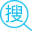# MATHEMATICA 学习笔记

## Software Note

WOLFRAM MATHEMATICA 实用编程指南 原书第二版

[美] 克里夫·黑斯廷斯 开尔文·米斯裘 迈克尔·莫里森著

## 特色

1. wolfram 语言指令以大写字母开头,若指令由多个单词组成，则每个单词的首字母都需要大写 (triks:自定义函数可以使用小写字母以便与内置函数相区别).
2. 函数参数用方括号 [] 括起.
3. 列表，也用于存储定义域和范围，用花括号 {} 括起来.

Example：Plot[Sin[x],{x,0,2$\pi$}]

**Manipulate[Plot[Sin[frequency\*x],{x,0,2$\pi$}],{frequency,1,5}]**


## PS：

#### 「Github登录用户留言」：